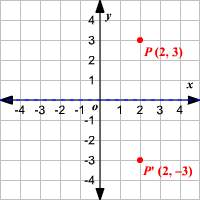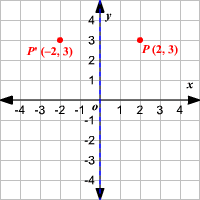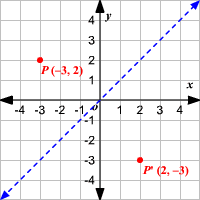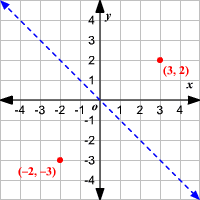# Reflections

A reflection is a transformation representing a flip of a figure. Figures may be reflected in a point, a line, or a plane. When reflecting a figure in a line or in a point, the image is congruent to the preimage.

A reflection maps every point of a figure to an image across a fixed line. The fixed line is called the line of reflection.

Some simple reflections can be performed easily in the coordinate plane using the general rules below.

### Reflection in the $x$ -axis:

A reflection of a point over the $x$ -axis is shown.The rule for a reflection over the $x$ -axis is $\left(x,y\right)\to \left(x,-y\right)$ .

### Reflection in the $y$ -axis:

A reflection of a point over the $y$ -axis is shown.The rule for a reflection over the $y$ -axis is $\left(x,y\right)\to \left(-x,y\right)$ .

### Reflection in the line $y=x$ :

A reflection of a point over the line $y=x$ is shown.The rule for a reflection in the line $y=x$ is $\left(x,y\right)\to \left(y,x\right)$

### Reflection in the line $y=-x$ :

A reflection of a point over the line $y=-x$ is shown.The rule for a reflection in the origin is $\left(x,y\right)\to \left(-y,-x\right)$ .